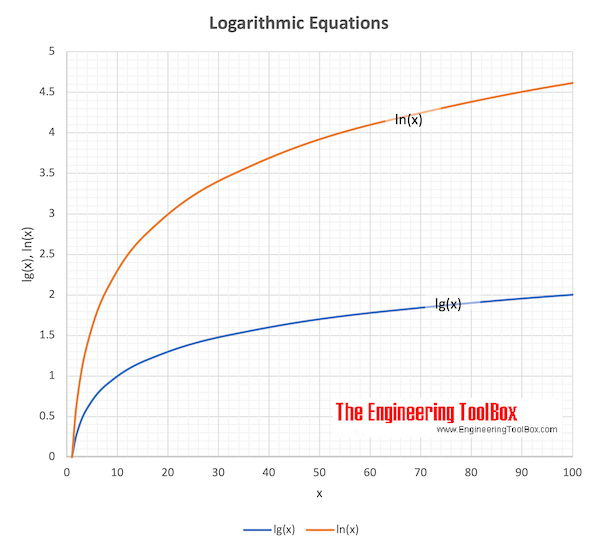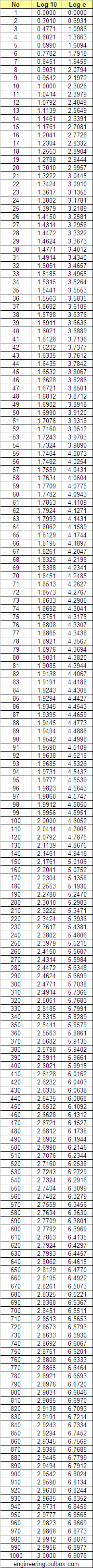Engineering ToolBox - Resources, Tools and Basic Information for Engineering and Design of Technical Applications!

# Logarithms

## The rules of logarithms - log10 and loge for numbers ranging 1 to 1000.

The logarithm (log) is the inverse operation to exponentiation - and the logarithm of a number is the exponent to which the base - another fixed value - must be raised to produce that number.

The expression

ay = x                                   (1)

can be expressed as the "base a logarithm of x" as

loga (x) = y                               (1b)

where

a = base

x = antilogarithm

y = logarithm (log)

#### Example - Logarithm with base 10

Since

103 = 1000

- then the base 10 logarithm of 1000 can be expressed as

log10 (1000) = 3

#### Natural Logarithm - Logarithm with base e (2.7182...)

ey = x

where

e = 2.7182.... - e constant or Euler's number

Base e logarithm of x can be expressed as

loge (x) = ln (x) = yLogarithms
SystemLog to the base ofTerminology
loga a log to base a
log10 = lg 10 common log
loge = ln e = 2.718281828459.. natural log
log2 = lb 2 log to base 2

### Rules for Logarithmic Calculations

loga (x y) = loga (x) + loga (y)                     (2)

loga (x / y) = loga (x) - loga (y)                   (3)

loga (xp) = p loga (x)                        (4)

loga (1 / x) = - loga (x)                        (5)

loga (b) = 1                        (6)

loga (1) = 0                        (7)

loga (0) = undefined                          (8)

loga (x < 0) = undefined                       (9)

loga (x) = logc (x) / logc (a)                     (10)

loga (x → ∞) =                             (11)

#### Example - Logarithm Product Rule

log10 ((5) (6)) = log10 (5) + log10(6)

= 0.6990 + 0.7782

= 1.4772

### Conversion of Logarithms

lg (x) = lg (e) ln (x)

= 0.434294 ln (x)                           (12)

ln (x) = lg (x) / lg (e)

= 2.302585 lg (x)                          (13)

lb (x) = 1.442695 ln (x)

= 3.321928 lg (x)                         (15)

### Log10 (x) and Loge (x)  for x ranging 1 to 1000## Related Topics

• ### Basics

The SI-system, unit converters, physical constants, drawing scales and more.
• ### Mathematics

Mathematical rules and laws - numbers, areas, volumes, exponents, trigonometric functions and more.

## Related Documents

• ### Algebraic Expressions

Principal algebraic expressions formulas.
• ### Amines, Diamines and Cyclic Organic Nitrogen Compounds - pKa Values

Values for the negative logarithm of the acid dissociation constant, pKa, of the conjugated acid of amines, diamines and cyclic organic nitrogen compounds, shown together with the molecular structure of the acids.
• ### Arithmetic and Logarithmic Mean Temperature Difference

Arithmetic Mean Temperature Difference in Heat Exchangers - AMTD - and Logarithmic Mean Temperature Difference - LMTD - formulas with examples - Online Mean Temperature Calculator.
• ### Constants of e

The mathematical e constants.
• ### Constants of PI

The mathematical π constants.
• ### Decibel

Logarithmic unit used to describe ratios of signal levels - like power or intensity - to a reference level.
• ### Exponents - Powers and Roots

The laws of fractional and integer exponents.
• ### Factorials

The product of all positive integers.
• ### Fractions

Law of fractions
• ### Inorganic Acids and Bases - pKa Values

Values for the negative logarithm of the acid dissociation constant, pKa, of inorganic acids and bases, as well as hydrated metal ions.
• ### Laws of Indices

Simplifying calculations by involving indices.
• ### Numerical Constants

Some numerical constants.
• ### pH - Basic (alkaline) vs. Acidic

Introduction to pH - the acidic and basic (alkaline) definition.
• ### Phenols, Alcohols and Carboxylic Acids - pKa Values

For oxygen containing organic compounds this is given: pKa (the negative logarithm of the acid dissociation constant), molecular structures, molar weights, density and melting and boiling points.
• ### Scientific and Engineering Terms - ANSI Abbreviations

Abbreviations for Use on Drawings and in Text - ANSI Y1.1.
• ### Signals - Adding Decibels

The logarithmic decibel scale is convenient when adding signal values like sound power, pressure and others from two or more sources.
• ### Solving Quadratic Equation with One Unknown

How to solve a quadratic equation.
• ### Sound Pressure

Sound Pressure is the force of sound on a surface perpendicular to the propagation of sound.
• ### Sound Propagation - the Inverse Square Law

Doubling of the distance from a noise source reduces the sound pressure level with 6 decibel.
• ### Trigonometric Functions

Sine, cosine and tangent - the natural trigonometric functions.

## Engineering ToolBox - SketchUp Extension - Online 3D modeling!

Add standard and customized parametric components - like flange beams, lumbers, piping, stairs and more - to your Sketchup model with the Engineering ToolBox - SketchUp Extension - enabled for use with older versions of the amazing SketchUp Make and the newer "up to date" SketchUp Pro . Add the Engineering ToolBox extension to your SketchUp Make/Pro from the Extension Warehouse !

We don't collect information from our users. More about

## Citation

• The Engineering ToolBox (2009). Logarithms. [online] Available at: https://www.engineeringtoolbox.com/logarithms-d_1246.html [Accessed Day Month Year].

Modify the access date according your visit.

10.2.10